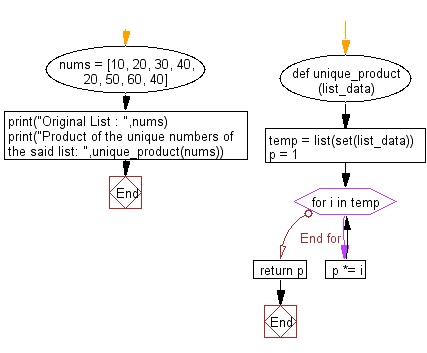﻿ Python: Calculate the product of the unique numbers of a given list - w3resource# Python: Calculate the product of the unique numbers of a given list

## Python List: Exercise - 125 with Solution

Write a Python program to calculate the product of the unique numbers of a given list.

Sample Solution:

Python Code:

``````def unique_product(list_data):
temp = list(set(list_data))
p = 1
for i in temp:
p *= i
return p
nums = [10, 20, 30, 40, 20, 50, 60, 40]
print("Original List : ",nums)
print("Product of the unique numbers of the said list: ",unique_product(nums))
```
```

Sample Output:

```Original List :  [10, 20, 30, 40, 20, 50, 60, 40]
Product of the unique numbers of the said list:  720000000
```

Flowchart:## Visualize Python code execution:

The following tool visualize what the computer is doing step-by-step as it executes the said program:

Python Code Editor:

Have another way to solve this solution? Contribute your code (and comments) through Disqus.

What is the difficulty level of this exercise?

Test your Python skills with w3resource's quiz

﻿

## Python: Tips of the Day

Floor Division:

When we speak of division we normally mean (/) float division operator, this will give a precise result in float format with decimals.

For a rounded integer result there is (//) floor division operator in Python. Floor division will only give integer results that are round numbers.

```print(1000 // 300)
print(1000 / 300)```

Output:

```3
3.3333333333333335```